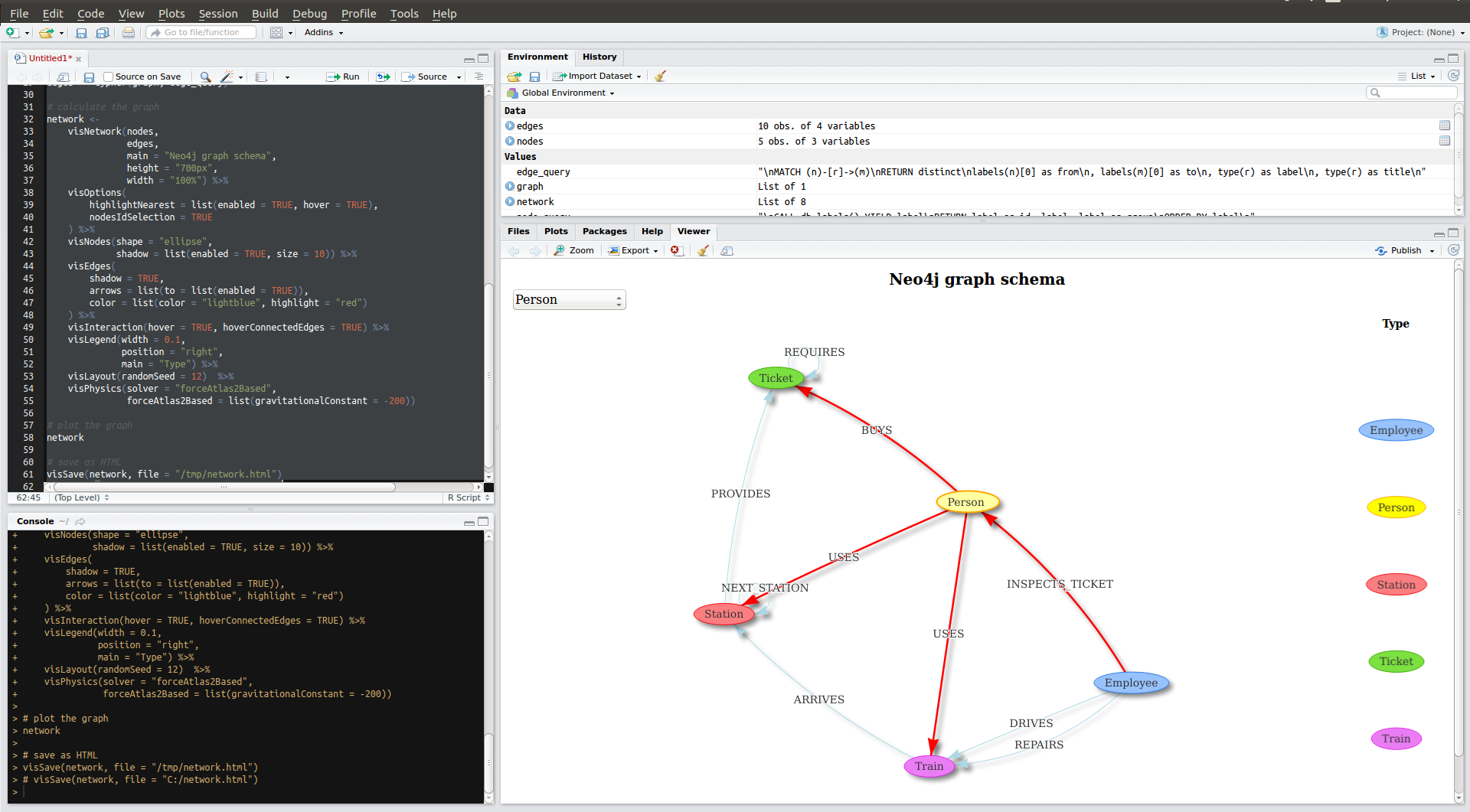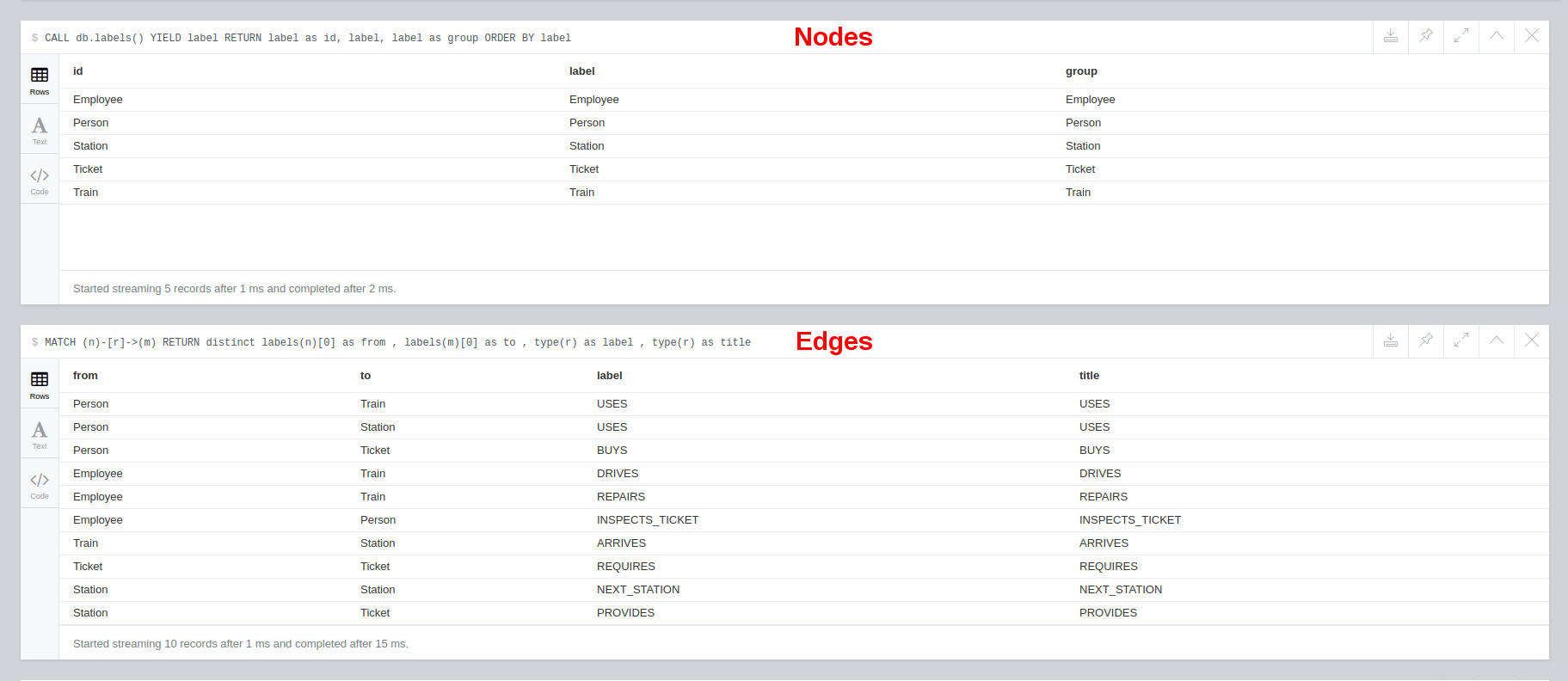# DeveloperA clever man solves a problem, a wise man avoids it.
— Albert Einstein

## Neo4j Schema visualization with R

The goal of this tutorial is to generate interactive HTML snippets for a prettier API documentation. »Example API documentation - Spring Data Neo4j«

# Preface

This tutorial uses RNeo4j a R package by Nicole White to visualize Metadata. Knowledge about the R programming language is NOT MANDATORY. I walk you through installing RStudio and executing R. You just need to know Cypher.

``````node_query <- "
CALL db.labels() YIELD label
RETURN label as id, label, label as group
ORDER BY label
"

edge_query <- "
MATCH (n)-[r]->(m)
RETURN distinct
labels(n) as from
, labels(m) as to
, type(r) as label
, type(r) as title
"
``````

# Requirements

## Demo database

### Local

1. Start a Neo4j server
2. If the database is empty copy-paste this example data → Neo4j Browser

### Docker

``````# start Neo4j database - https://hub.docker.com/_/neo4j/
docker run -ti --rm  \
-p 7474:7474 \
-p 7687:7687 \
--env=NEO4J_AUTH=none \
phoen1x/visnetwork-graph-schema
``````

## Setup RStudio

### Install RStudio

Watch this video - Install RStudio

### Install required Packages

Watch this video - Install Packages

``````install.packages("visNetwork",repos = "http://cran.r-project.org")
install.packages("RNeo4j",repos = "http://cran.r-project.org")
``````

# Source Code

### R-Script

Start RStudio and open a new R-Script tab Ctrl+Shift+N. Then copy-paste the source code. View on GitHub

``````# http://datastorm-open.github.io/visNetwork/
library(visNetwork)

# https://github.com/nicolewhite/RNeo4j
library(RNeo4j)

# connect to database
graph <- startGraph("http://localhost:7474/db/data/")

# https://nicolewhite.github.io/2015/06/18/visualize-your-graph-with-rneo4j-and-visNetwork.html
node_query <- "
CALL db.labels() YIELD label
RETURN label as id, label, label as group
ORDER BY label
"

edge_query <- "
MATCH (n)-[r]->(m)
RETURN distinct
labels(n) as from
, labels(m) as to
, type(r) as label
, type(r) as title
"

# get neo4j data
nodes <- cypher(graph, node_query)
edges <- cypher(graph, edge_query)

# calculate the graph
network <-
visNetwork(nodes,
edges,
main = "Neo4j graph schema",
height = "700px",
width = "100%") %>%
visOptions(
highlightNearest = list(enabled = TRUE, hover = TRUE),
nodesIdSelection = TRUE
) %>%
visNodes(shape = "ellipse",
shadow = list(enabled = TRUE, size = 10)) %>%
visEdges(
arrows = list(to = list(enabled = TRUE)),
color = list(color = "lightblue", highlight = "red")
) %>%
visInteraction(hover = TRUE, hoverConnectedEdges = TRUE) %>%
visLegend(width = 0.1,
position = "right",
main = "Type") %>%
visLayout(randomSeed = 12)  %>%
visPhysics(solver = "forceAtlas2Based",
forceAtlas2Based = list(gravitationalConstant = -200))

# plot the graph
network

# save as HTML
visSave(network, file = "/tmp/network.html")
# visSave(network, file = "C:/network.html")
``````

Mark all lines Ctrl+A and run the R-Script Ctrl+Enter

## Result## Troubleshoot

You can watch more of MarinStatsLectures if you need help executing R-Scripts.

#### Troubleshoot Windows

``````# change value
visSave(network, file = "C:/network.html")
``````

#### Troubleshoot URL and authentication

``````# change values
``````

## How it works## Tweak result

You probably want to customize the node_query / edge_query and explore your own schema with the Neo4j procedures

``````# "native" neo4j schema call
CALL db.schema()

# get all node types
CALL db.labels()

# get all edge types
CALL db.relationshipTypes()
``````

Or add restrict the nodes to view only a part of the graph.

``````node_query <- "
CALL db.labels() YIELD label
WHERE label IN ['Person','Station','Train']
..."

edge_query <- "
MATCH (n)-[r]->(m)
WHERE labels(n) IN ['Person','Station','Train']
..."
``````

Or tweak the graph layout with the visNetwork documentation

``````# repulsion of nodes -> length of edges
forceAtlas2Based = list(gravitationalConstant = -50)
``````

# Sample data``````# http://datastorm-open.github.io/visNetwork/
library(visNetwork)

# https://github.com/nicolewhite/RNeo4j
library(RNeo4j)

# connect to database
graph <- startGraph("http://localhost:7474/db/data/")

# https://nicolewhite.github.io/2015/06/18/visualize-your-graph-with-rneo4j-and-visNetwork.html
node_query <- "
MATCH (n)
WHERE n.name in ['Alice','Bob','Express train','Subway','Ticket Zone1','Ticket Zone3','First Class Travel','Station North']
RETURN  n.name as id
, n.name as label
, labels(n) as group
"

edge_query <- "
MATCH (n:Person)-[r]->(m)
WHERE n.name IN ['Alice','Bob']
RETURN
n.name as from
, m.name as to
, type(r) as label
, type(r) as title
UNION
MATCH (n:Ticket{name:'First Class Travel'})-[r]->(m:Ticket{name:'Ticket Zone3'})
RETURN
n.name as from
, m.name as to
, type(r) as label
, type(r) as title
UNION
MATCH (n:Train)-[r]->(m:Station{name:'Station North'})
RETURN
n.name as from
, m.name as to
, type(r) as label
, type(r) as title
"

# get neo4j data
nodes <- cypher(graph, node_query)
edges <- cypher(graph, edge_query)

# calculate the graph
network <-
visNetwork(nodes,
edges,
main = "Sample: First Class Travel",
height = "700px",
width = "100%") %>%
visOptions(
highlightNearest = list(enabled = TRUE, hover = TRUE),
nodesIdSelection = TRUE
) %>%
visNodes(shape = "ellipse",
shadow = list(enabled = TRUE, size = 10)) %>%
visEdges(
arrows = list(to = list(enabled = TRUE)),
color = list(color = "lightblue", highlight = "red")
) %>%
visInteraction(hover = TRUE, hoverConnectedEdges = TRUE) %>%
visLegend(width = 0.1,
position = "right",
main = "Type") %>%
visGroups(groupname = "Person", color = "#FFFB4F") %>%
visGroups(groupname = "Station", color = "#FF7D81") %>%
visGroups(groupname = "Ticket", color = "#69DD5B") %>%
visGroups(groupname = "Train", color = "#F382ED") %>%
visLayout(randomSeed = 12)  %>%
visPhysics(solver = "forceAtlas2Based",
forceAtlas2Based = list(gravitationalConstant = -80))

# plot the graph
network

# save as HTML
visSave(network, file = "/tmp/network.html")
# visSave(network, file = "C:/network.html")
``````

# Docker

Make sure you have a working Docker, docker-compose and Git environment.

``````# download project
git clone https://github.com/phoen1x/visnetwork-graph-schema.git

# start container
cd visnetwork-graph-schema/docker
docker-compose up -d

# WAIT A FEW SECONDS for Neo4j to boot then run R-Scripts
docker-compose exec neo4j Rscript /scripts/Docker.R &> /dev/null

# view results in web browser
firefox ../scripts/output/Docker.html

# stop container
docker-compose down
``````

# Beyond R and visNetwork

## vis.js the Javascript library behind visNetwork

Define what you want to plot in DOT language

``````var DOTstring = 'mynetwork {';
DOTstring +=    '    Person->Station;'
DOTstring +=    '    Person->Train [label=USES];'
DOTstring +=    '    Station->Train [label=ARRIVES];'
DOTstring +=    '}'
``````

And use this HTML Template to import DOT language into vis.js

``````<!doctype html>
<html>
<title>Your Neo4j database state --- Sat Apr 01 12:34:56 CEST 2017</title>
<script type="text/javascript" src="https://cdnjs.cloudflare.com/ajax/libs/vis/4.21.0/vis.min.js"></script>
<style type="text/css">
#mynetwork {
width: 600px;
height: 400px;
border: 1px solid lightgray;
}
</style>
<body>
<p>Your Neo4j database state --- Sat Apr 01 12:34:56 CEST 2017</p>
<div id="mynetwork"></div><div id="config"></div>
<script type="text/javascript">
// provide data in the DOT language
var DOTstring = 'mynetwork {';
DOTstring +=    '    Employee -> Person [label=INSPECTS_TICKET];';
DOTstring +=    '    Employee -> Train [label=DRIVES];';
DOTstring +=    '    Employee -> Train [label=REPAIRS];';
DOTstring +=    '    Person -> Station [label=USES];';
DOTstring +=    '    Person -> Ticket [label=BUYS];';
DOTstring +=    '    Person -> Train [label=USES];';
DOTstring +=    '    Station -> Ticket [label=PROVIDES];';
DOTstring +=    '    Ticket -> Ticket [label=REQUIRES];';
DOTstring +=    '    Train -> Station [label=ARRIVES];';
DOTstring +=    '}'

var parsedData = vis.network.convertDot(DOTstring);
//parsedData.nodes.color='yellow';

for (var i = 0; i < parsedData.nodes.length; i++) {
parsedData.nodes[i].group = parsedData.nodes[i].label;
}

parsedData.options = {
nodes: {
shape: 'ellipse',
borderWidth: 2,
},
edges: {
width: 2,
smooth: {
forceDirection: "none"
}
},
"physics": {
"forceAtlas2Based": {
"springLength": 100
},
"minVelocity": 0.75,
"solver": "forceAtlas2Based"
},
configure: {
filter:function (option, path) {
if (path.indexOf('physics') !== -1) {
return true;
}
if (path.indexOf('smooth') !== -1 || option === 'smooth') {
return true;
}
return false;
},
container: document.getElementById('config')
}
};

// create a network
var network = new vis.Network(
document.getElementById('mynetwork'), {
nodes: parsedData.nodes,
edges: parsedData.edges
},
parsedData.options);
</script>
</body>
</html>
``````

## Spring Data Neo4j

### Graph schema

Spring Data Neo4j provides the @Relationship annotation

``````public class Person {
direction = Relationship.OUTGOING)
private List<Ticket> tickets;
}
``````

which can be analyzed via Java Reflection and translated to DOT.

### Build documentation

``````# download project
git clone https://github.com/phoen1x/visnetwork-graph-schema.git

# build jar / documentation
cd visnetwork-graph-schema/java/visnetwork-graph-schema
./mvnw clean package

# open result in web browser
firefox target/docs/index.html
``````

### Show database state

``````./mvnw surefire:test -Dtest=DotNotationPrinterTest

# open result HTML
firefox target/snippetGraphOverview.html
``````

### Important source code

Be aware that the GitHub example is only a quick and dirty prototype to see if it is feasible to automatically generate snippets. For proper implementation see:

# GitHub / DockerHub / Comments

Please improve this tutorial and leave your comments in the GitHub issue section. Also check the DockerHub repository if you want to know more about the demo container.